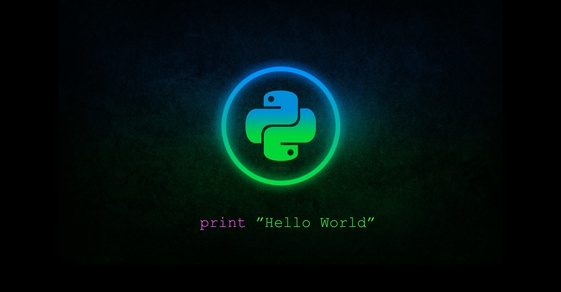Aprann kreye sit wèb ak Django, fasil epi byen rapid... Learn more.

## How To Create A Simple Program With Python As A Beginner

Last update: Oct. 12, 2021, 7:48 p.m.
Tags :
Duration : 30 min
Views : 2692
Available in : Haitian CreoleIn this tutorial, I will teach python beginners how to create a simple program, where you will have to interact with the users to ask them numbers and to display which number is greater. This program will be easy to implement, but useful to help you master the basics of Python.

Python is easy, if you have decided to learn it, that's great. As you can notice across the Internet, many people will advise you to start with Python. So I also advise you to do so - let's start.

Our program will ask the user to provide two numbers. With these two numbers, we will determine the greater and print it out. The built-in function to do that in python is `input("prompt_message")` function that allows user input. The `prompt_message` is a String, representing a default message before the input.

[class=language-python]

``````# my awesome program
number1 = input("Enter the first number: ")
``````

Now we have the first number, we need to make sure that the user enters a correct number. Never trust users in your project. To do that, we must check whether it is actually digits, with a method inherited from all string class in Python called `isdigit()`. Remember that we need to keep asking the user to enter the correct value with a loop. The loop to use here is `while`.

[class=language-python]

``````# my awesome program
number1 = input("Enter the first number: ")
while (not number1.isdigit()):  # while the number is not a digit
number1 = input("Please, enter the first number (digits)")
``````

To avoid repeating the same block of functionalities in our project, we are going to use a function to do the same thing for the second number. Our program will be like the following.

[class=language-python]

``````# my awesome program
def customInput(prompt_message):
num = input(prompt_message)
while (not num.isdigit()):  # while the number is not a digit
num = input("Please, %s (digits)" % prompt_message)
return num

number1 = customInput("Enter the first number: ")
number2 = customInput("Enter the second number: ")
``````

We created a function to a little bit empower our program. Let me explain what I did:

• `def customInput(prompt_message):` I declare the function with the keyword `def` and I give a name to it `customName`. You are free to name it as you want. Make sure your names don't begin with numbers, don't contain special characters except underscore(_), don't have the same name as python built-in functions

• `num = input(prompt_message)`, I use the same python built-in function I pass as parameter, the parameter coming from the function `customInput()`

• `num = input("Please, %s (digits)" % (prompt_message))` In that line, I format the string with `%s`, the first `%s` will be replaced with by the first value found which is `prompt_message`

Since everything coming from `input()` is string, we need to convert the value received to integer. Change the last line of your function like the following: `return int(num)`. `int()` is a function that converts values to integer.

There's nothing else to do than testing which number is greater with conditions: `if`, `elif`, `else`

[class=language-python]

``````# my awesome program
def customInput(prompt_message):
num = input(prompt_message)
while (not num.isdigit()):  # while the number is not a digit
num = input("Please, %s (digits)" % prompt_message)
return int(num)

number1 = customInput("Enter the first number: ")
number2 = customInput("Enter the second number: ")

if number1 > number2:
print("The first number is greater than the second")
elif number1 < number2:
print("The second number is greater than the first")
else:
print("Those numbers are same")
``````

And that's it, we are done with the program, you can test it. This program will help you master some basic concepts of Python. Please email me at lemayzeur@code9haiti.com if drop a comment below if you want to learn more about Python.#### by Lub Lorry Lamysere on Mar. 22, 2019

Software Developer | Entrepreneur###### Maxime Milien3 years, 2 months ago

mwen ta swete yon lhr pou code9 vinn pi gro platform in Hait e mwen santi sa pa lwe rive
avec css HTML sa ou ta mete yon kou PHP frem ou tap fem renmn site sa net